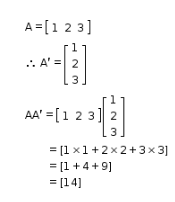# If matrix A = [1 2 3], write AA', where A' is the transpose of matrix A

If matrix A = [1 2 3], write AA’, where A’ is the transpose of matrix A.